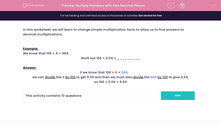# Multiply Numbers with Two Decimal Places

In this worksheet, students practise decimal multiplications to two places given existing relevant multiplication facts.Key stage:  KS 2

Curriculum topic:   Maths and Numerical Reasoning

Curriculum subtopic:   Decimals

Difficulty level:#### Worksheet Overview

In this worksheet, we will learn to change simple multiplication facts to allow us to find answers to decimal multiplications.

Example:

We know that 166 × 4 = 664.

Work out 166 × 0.04 = _________

If we know that 166 × 4 = 664,

we can divide the 4 by 100 to get 0.04 and then we must also divide the 664 by 100 to give 6.64,

so 166 × 0.04 = 6.64

### What is EdPlace?

We're your National Curriculum aligned online education content provider helping each child succeed in English, maths and science from year 1 to GCSE. With an EdPlace account you’ll be able to track and measure progress, helping each child achieve their best. We build confidence and attainment by personalising each child’s learning at a level that suits them.

Get started